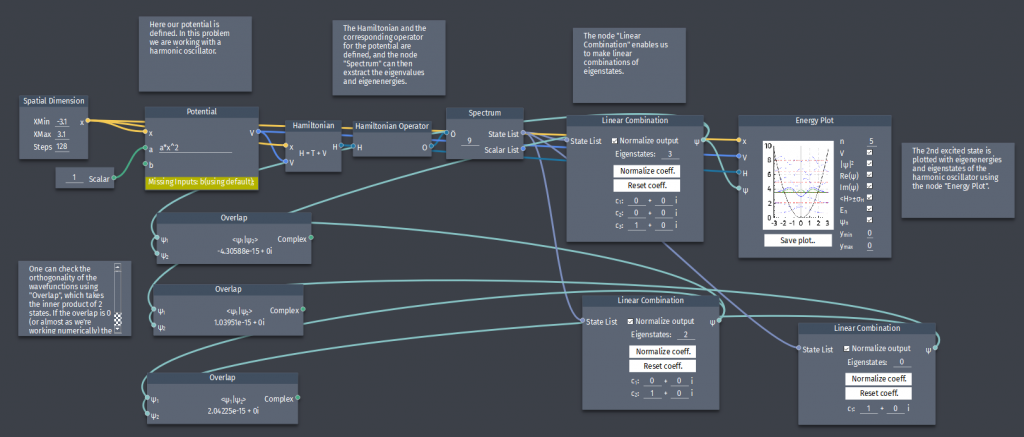# Eigenstates of the harmonic oscillator

In this problem we are studying the harmonic oscillator, i.e. the potential $V(x)=\frac{1}{2}m\omega^2 x^2$.

a) Construct the 2nd excited state $\psi_{2}(x)$.

b) Plot the ground state $\psi_{0}(x)$, the 1st and 2nd excited state ($\psi_{1}(x),\psi_{2}(x))$.

c) Check the orthogonality of the eigenfunctions.